# Summary of simple and easy ways to calculate the average score in Excel

Excel is known as a software that gives people a lot of useful features for calculations, in which we cannot ignore the feature of calculating the average score with maximum accuracy. Please refer to the simple way to calculate the average score in Excel shared by Sforum below!## Use the Average function to calculate the average score in Excel

Syntax of the function: =Average(number1,[number2],…).

Step 1: You enter the Average function in the cell where you want to get the average score result.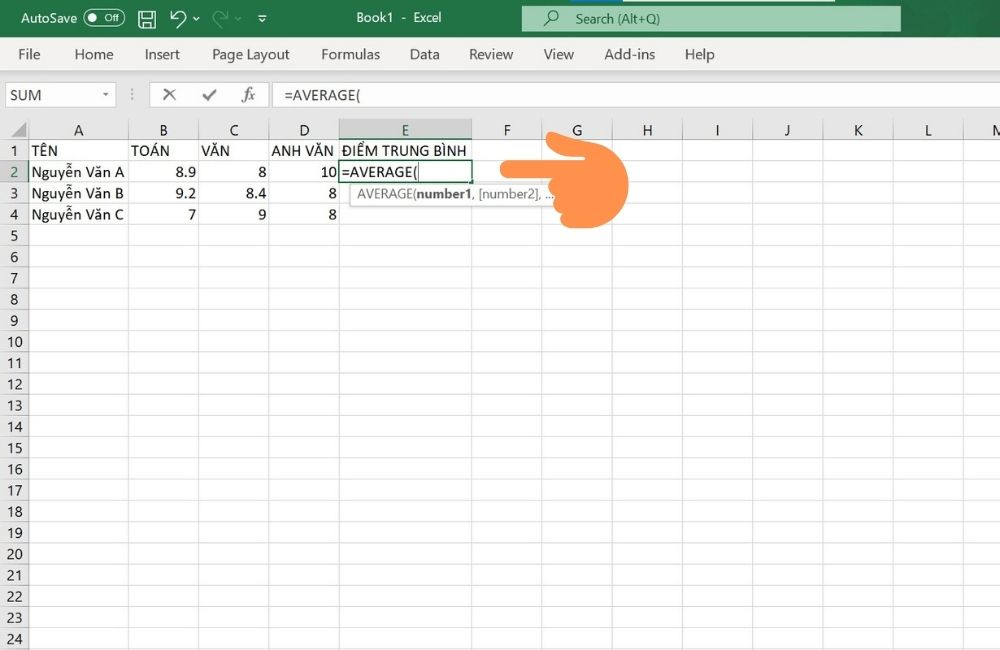Step 2: Friend mouse click in the cells where you want to calculate the average score or drag the mouse from the first cell to the last cell of the data range for which you want to calculate the average score.

Specifically as in the pictures below, if you want to calculate the average score of cells from B2, C2, D2, you can enter the following syntax: “=AVERAGE(B2,C2,D2)”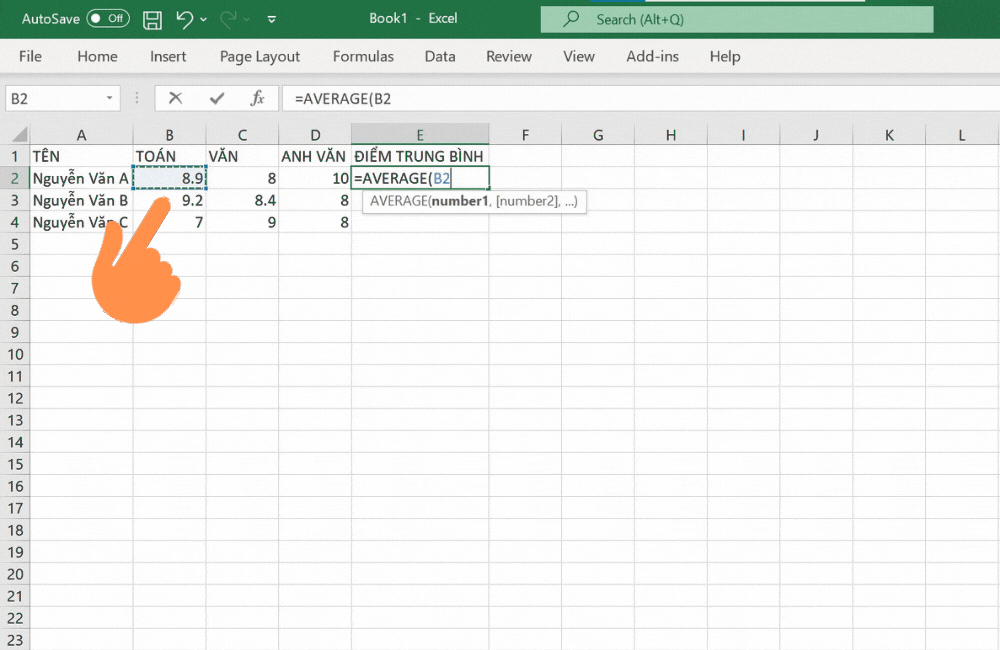Step 3: Press Enter to get results.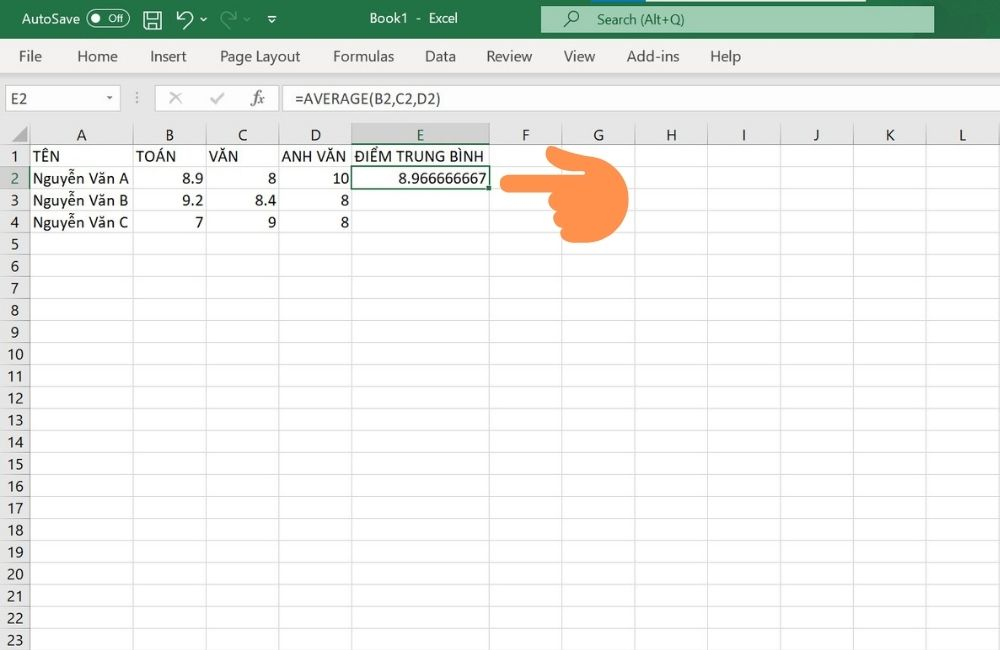## Use the average formula to calculate the average score in Excel

### Calculate grade point average without coefficients

Step 1: If you want to calculate the average of 3 columns of regular, midterm, and final grades, enter the syntax “=(B2+C2+D2)/3”.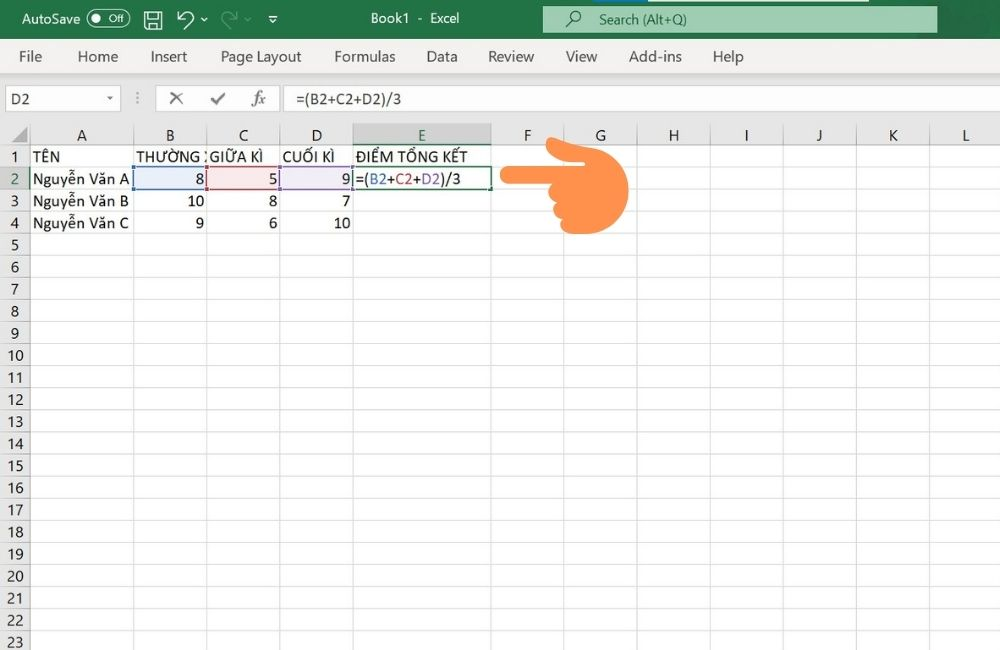Step 2: Press Enter to get the results.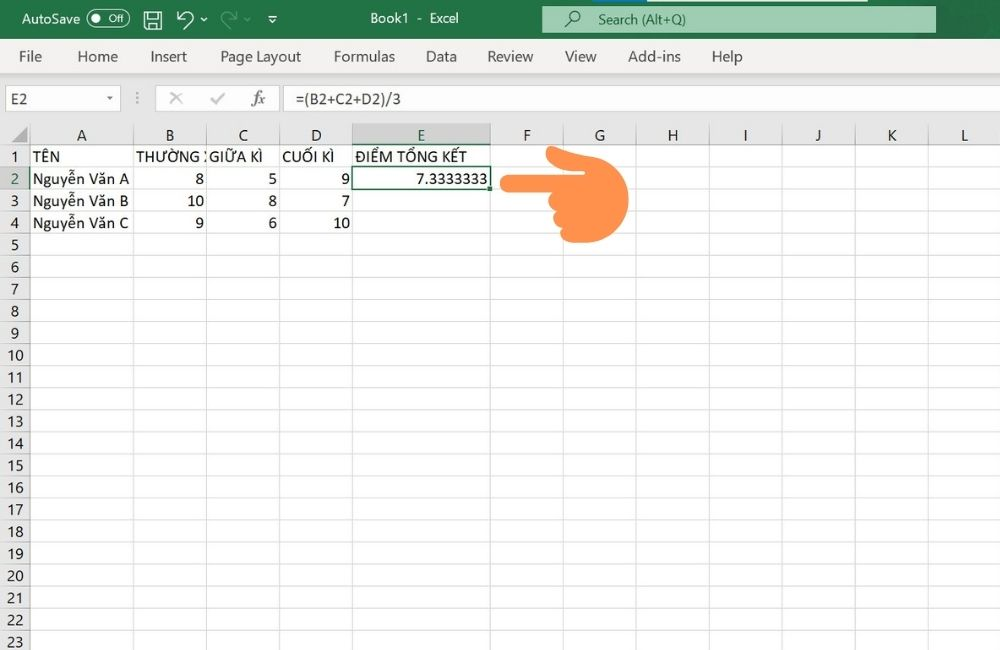### Calculate mean score with coefficient

Step 1: If you want to calculate the average of the 3 grades, but multiply by a factor of 2 for the midterm score and multiply by 3 for the final grade, enter the syntax “=(B2+C2*2+D2*3)/6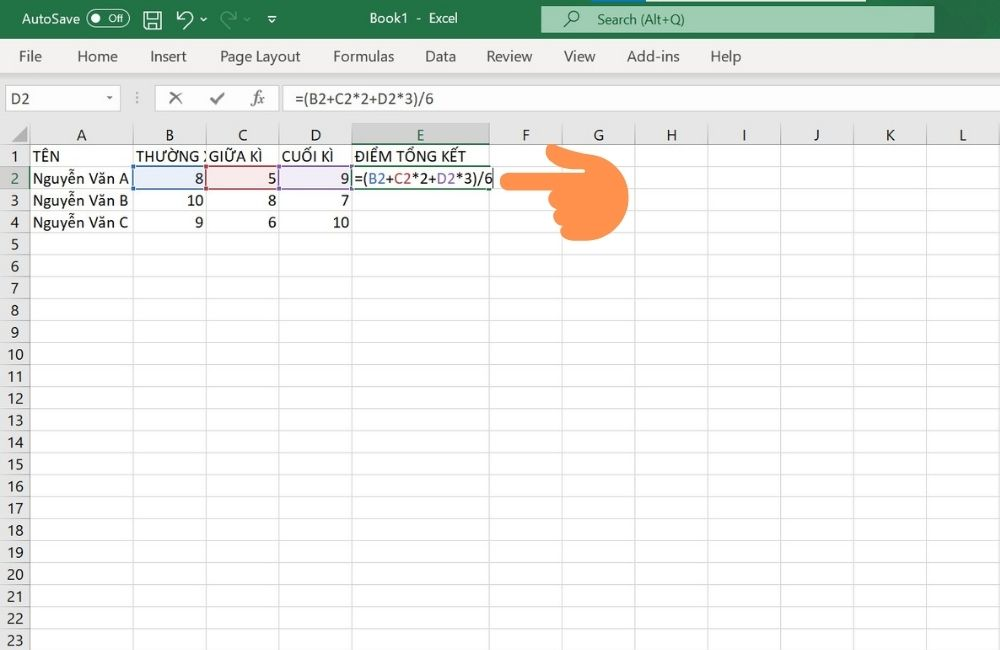Step 2: Press Enter to get results.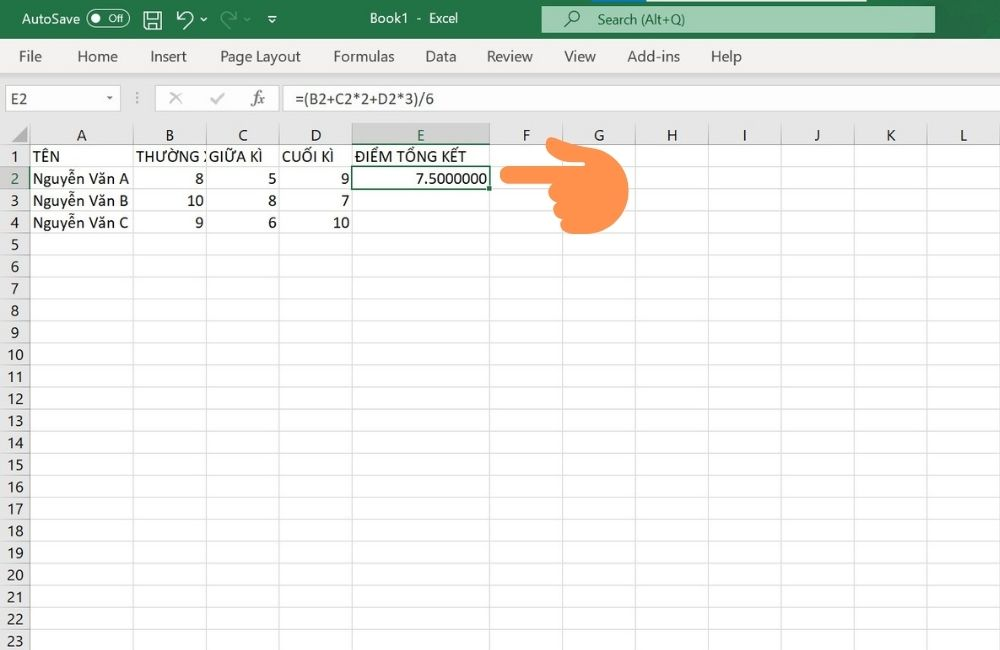Note: After calculating the score, if the decimal point after the comma is too long, you can quickly round the result at the icon as shown below.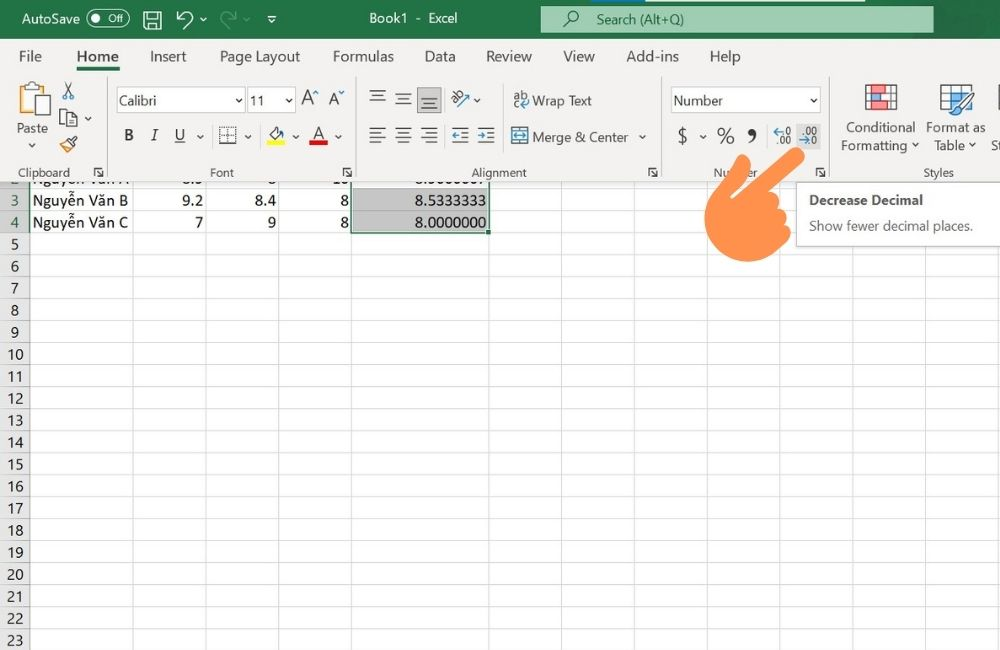## Ending

Above are 2 simple and easy ways to calculate the average score in Excel that Sforum wants to share with you. Wish you successful practice and leave a comment below the article if you have any questions in the steps!

Published